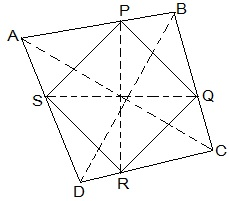Guru

# Show that the line segments joining the mid-points of the opposite sides of a quadrilateral bisect each other. Q.6

• 0

Please give me the best way for solving the problem of class 9th ncert math of Quadrilaterals chapter of math of class 9th of exercise 8.2 of question no 6 what is the tricky way for solving this question Show that the line segments joining the mid-points of the opposite sides of a quadrilateral bisect each other.

Share

1. :Let ABCD be a quadrilateral and P, Q, R and S are the mid points of AB, BC, CD and DA respectively.

Now,

In ΔACD,

R and S are the mid points of CD and DA respectively.

, SR || AC.

Similarly we can show that,

PQ || AC,

PS || BD and

QR || BD

, PQRS is parallelogram.

PR and QS are the diagonals of the parallelogram PQRS. So, they will bisect each other.

• 0